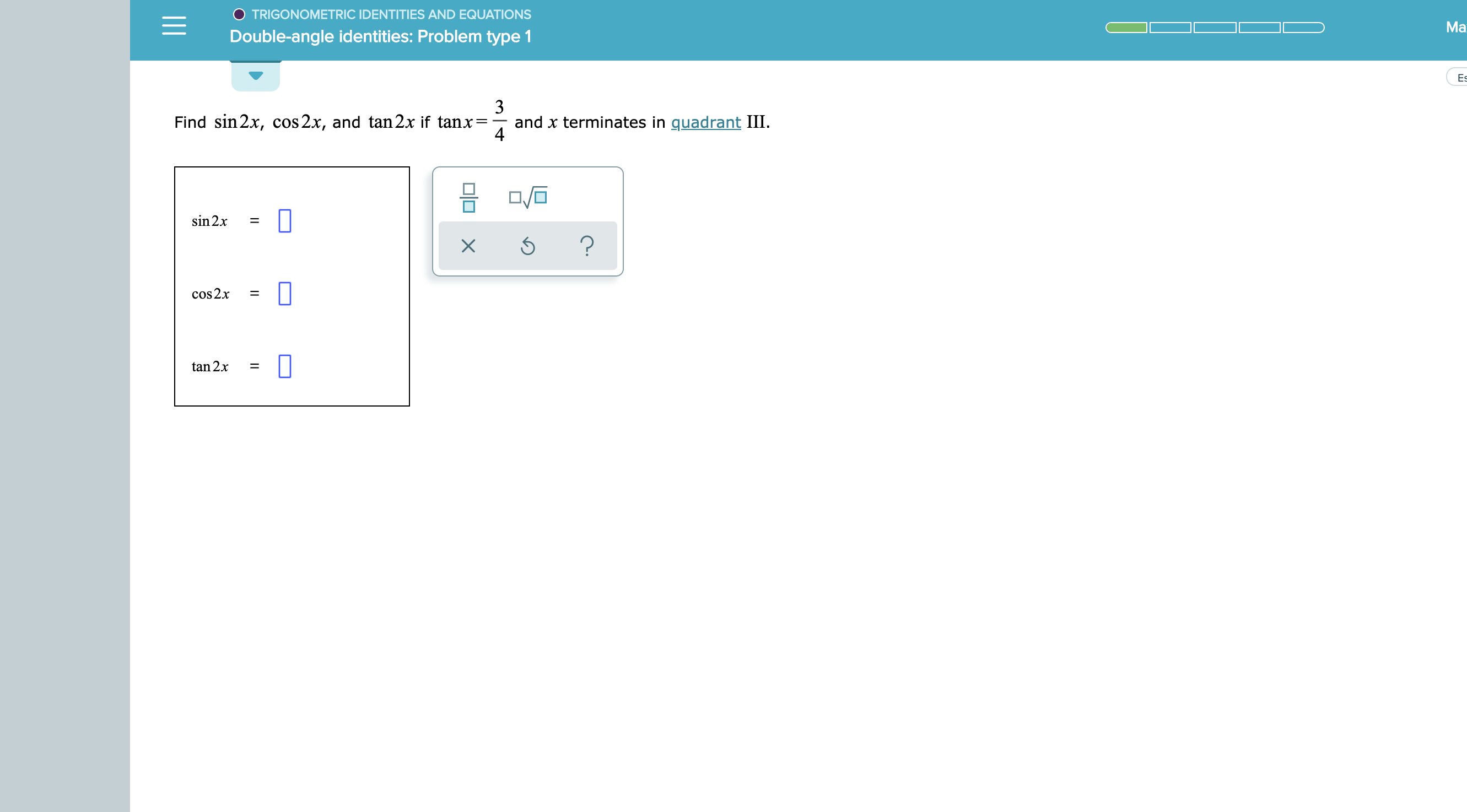# O TRIGONOMETRIC IDENTITIES AND EQUATIONSMaDouble-angle identities: Problem type 1Es3Find sin 2x, cos 2x, and tan2x if tanx=and x terminates in quadrant III4sin 2x?cos2xtan 2x=X

Question
1 views

see attachmenthelp_outlineImage TranscriptioncloseO TRIGONOMETRIC IDENTITIES AND EQUATIONS Ma Double-angle identities: Problem type 1 Es 3 Find sin 2x, cos 2x, and tan2x if tanx= and x terminates in quadrant III 4 sin 2x ? cos2x tan 2x = X fullscreen
check_circle

Step 1

Given,

Step 2

On solving the above equation, we get

Step 3

Since, x lies in the 3rd q...

### Want to see the full answer?

See Solution

#### Want to see this answer and more?

Solutions are written by subject experts who are available 24/7. Questions are typically answered within 1 hour.*

See Solution
*Response times may vary by subject and question.
Tagged in

### Trigonometric Ratios